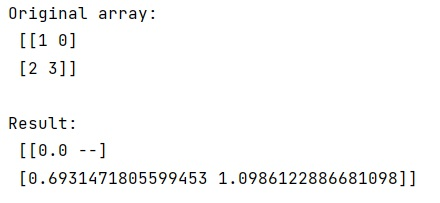# NumPy: Efficiently avoid 0s when taking log (matrix)

Compute log2() of elements of a numpy array where the element is not zero?
Submitted by Pranit Sharma, on February 15, 2023

NumPy is an abbreviated form of Numerical Python. It is used for different types of scientific operations in python. Numpy is a vast library in python which is used for almost every kind of scientific or mathematical operation. It is itself an array which is a collection of various methods and functions for processing the arrays.

## Avoiding 0s when taking log (matrix)

Suppose that we are given a numpy array that contains some numeric values including 0. We need to compute element-wise log2() but not in the case where the element is 0.

For this purpose, we can use the numpy.where clause and it will work fine but it will give us a warning for dividing by zero.

Hence, we need to work on a masked array, we will create a mask of the numpy array and then calculate the log on each element.

Let us understand with the help of an example,

## Python code for efficiently avoid 0s when taking log (matrix) in numpy

```# Import numpy
import numpy as np

# Creating a numpy array
arr = np.array([[1,0], [2,3]])

# Display original array
print("Original array:\n",arr,"\n")

# Creating a mask and computing log
res = np.ma.log(arr)

# Display result
print("Result:\n",res)
```

Output: